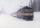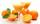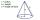# Ratio

The radii of two cones are in the ratio 5.7
Calculate the area ratio if cones have same height.

Result

x =  0

Leave us a comment of example and its solution (i.e. if it is still somewhat unclear...):Be the first to comment!## Next similar examples:

1. MedianThe median of the triangle LMN is away from vertex N 84 cm. Calculate the length of the median, which start at N.
2. Percentage 1052 shorts and missed 13. Calculate percentage
3. TrainThe train passes part of the line for 95 minutes at speed 75 km/h. What speed would have to go in order to shorten the driving time of 20 minutes?
4. Cross-sections of a coneCone with base radius 16 cm and height 11 cm divide by parallel planes to base into three bodies. The planes divide the height of the cone into three equal parts. Determine the volume ratio of the maximum and minimum of the resulting body.
5. AnglesIn the triangle ABC, the ratio of angles is: a:b = 4: 5. The angle c is 36°. How big are the angles a, b?
6. RatioIncrease in the ratio 20:4 number 18.5.
7. SequenceFind the common ratio of the sequence -3, -1.5, -0.75, -0.375, -0.1875. Ratio write as decimal number rounded to tenth.
8. Ratio v2Decrease in the ratio 12:16 number 13.2.
9. Ratio 11Simplify this ratio 10 : 1/4
10. Bottles of juiceHow many 2-liter bottles of juice need to buy if you want to transfer juice to 50 pitchers rotary cone shape with a diameter of 24 cm and base side length of 1.5 dm.
11. Cone area and sideCalculate the surface area and volume of a rotating cone with a height of 1.25 dm and 17,8dm side.
12. Truncated coneA truncated cone has a bases radiuses 40 cm and 10 cm and a height of 25 cm. Calculate its surface area and volume.
13. Truncated coneCalculate the volume of a truncated cone with base radiuses r1=13 cm, r2 = 10 cm and height v = 8 cm.
14. Rotating coneCalculate volume of a rotating cone with base radius r=12 cm and height h=7 cm.
15. Cube surface areaWall of cube has content area 99 cm square. What is the surface of the cube?
16. KitchenKitchen roller has a diameter 70 mm and width of 359 mm. How many square millimeters roll on one turn?
17. The diagramThe diagram is a cone of radius 8cm and height 10cm. The diameter of the base is. ..